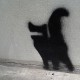Election Stats

January 14, 2008

Raw Data for NH Primary – DIY stats

Filed under: diebold, new hampshire — Brian @ 12:14 pm

This post will keep track of any work that I have done, and anyone is welcome to use it and do a better job than me.

Here is a spreadsheet with the vote totals and counting methods:

(it has been updated a few times, the earliest version had errors based upon my source. I wrote a script to parse the data from http://checkthevotes.com/. A few days ago, when that data was hosted at http://ronrox.com, this page was much simpler to parse. Now it is a bit of a mess. The reason I chose this site is because it had all the results and counting methods on one page. He grabbed his data from politico.com).

Because of discrepancies between the checkthevotes and the NH SOS website (nearly all the towns have +/- 5 vote count differences and there are/were 19 incorrect hand/Diebold misassignments), I wrote a script to parse the ugly HTML files containing the official results. I added a new worksheet to my Google spreadsheet above, and also made the output available on my box.net account (so far, just the Dems). I will post the script at some point if you want to check it.

If you want that data usable in R, just save that spreadsheet as a tab delimited file.
If you want to make a map of New Hampshire using R, you need the shapefile from the US Census: http://www.census.gov/geo/www/cob/cs2000.html#shp

Here is the R code I used generate maps and calculate a few linear models:

```# install the right packages
install.packages(c("maptools", "maps"), dependencies=T))

# get the vote data

# get the map data
library(maptools)

# get the lat/lon for each town
centroids <- get.Pcent(nhshp)
cents <- data.frame(CS33_D00_I=nhshp\$att.data\$CS33_D00_I, long=centroids[,1], lat=centroids[,2])
cents <- data.frame(cents, se_dist=((71+cents\$long)**2 + (cents\$lat-42)**2)**.5)

# add the centroid lat/lon and distance from SE corner to the map data
map_data <- merge(nhshp\$att.data, cents, all.x=T)

# convert the map names
names <- sub(names, pattern=" City", replacement="")
names <- sub(names, pattern=" Township", replacement="")
names <- sub(names, pattern=" Town", replacement="")
map_data\$NAME <- names

# merge the vote info with the map data (make sure it is sorted by the same key that
# the original map data is sorted by so that the subsetting works ok)
tmp <- merge(map_data, nhv2, all.x=T)
map_data <- tmp[sort.list(tmp\$CS33_D00_I),]

# make a map object
mp <- Map2poly(nhshp)

# make a plot -  color by method
plot(mp,forcefill=FALSE)
plot(subset(mp, map_data\$method == "Hand"), col="yellow",add=T, forcefill=F)
plot(subset(mp, map_data\$method == "Diebold"), col="brown",add=T, forcefill=F)

# make a plot - color by distance
plot(mp,forcefill=FALSE)
plot(subset(mp, map_data\$se_dist < 1), col="blue",add=T, forcefill=F)
plot(subset(mp, map_data\$se_dist >= 1 & map_data\$se_dist < 2 ), col="purple",add=T, forcefill=F)
plot(subset(mp, map_data\$se_dist >= 2 ), col="red",add=T, forcefill=F)

# color by town size
plot(mp,forcefill=FALSE)
plot(subset(mp, map_data\$dem_size >= 800 ), col="lightblue",add=T, forcefill=F)
plot(subset(mp, map_data\$dem_size >= 500 & map_data\$dem_size < 800 & map_data\$method == "Diebold"), col="purple",add=T, forcefill=F)
plot(subset(mp, map_data\$dem_size >= 500 & map_data\$dem_size < 800 & map_data\$method == "Hand"), col="blue",add=T, forcefill=F)
plot(subset(mp, map_data\$dem_size < 500 ), col="grey",add=T, forcefill=F)

# calculate the linear model and anova
l <- lm(map_data\$cliv ~ map_data\$dem_size + map_data\$method)
anova(l)

l <- lm(map_data\$cliv ~ map_data\$dem_size + map_data\$method + map_data\$se_dist + map_data\$lat)
anova(l)

# latitude and se_dist are the most important variables in determining the method ?
l <- lm(map_data\$se_dist ~ map_data\$method + map_data\$cliv + map_data\$dem_size + map_data\$lat)
anova(l)```

1. […] [Finally, if you want to repeat this analysis, please feel free to use the data and code that I have used] […]

Pingback by Vote Counting methods, drawn on a NH map « Election Stats — January 14, 2008 @ 1:04 pm

2.Some of it may be corrected at this point, but please note that ronrox.com/checkthevotes.com had incomplete/incorrect data both for which towns use Diebold and for vote counts. I suggest using the data from the nh.gov site.

Example of problems– Campton and several other towns are Diebold according to nh.gov, you have them as hand count. checkthevotes.com has 0 votes for at least one town, which conflicts with nh.gov.

Comment by John — January 14, 2008 @ 5:36 pm

3.I fixed my data according to your sources… You’re right! it was not accurate.

Comment by Brian — January 27, 2008 @ 11:36 pm